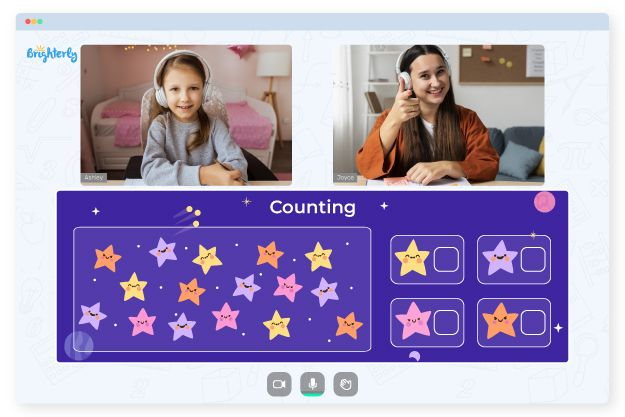# Ten Frame Worksheets

When a child first encounters ten frames in class, they research two by five rectangles so that counters can be placed in them to show numbers less than or equal to 10. The ten frames help kids understand the numbers within the context of 10; youngsters learn to subtract and add numbers within ten using the ten frame. So, ten frame math worksheets are incredibly beneficial to students in this regard.

## Benefits of ten frames worksheets

The benefits of 10 frame worksheets are numerous, and here are a few:

• Ten frame worksheets help students understand addition and subtractions within the number 10 using the ten frame boxes.
• Tens frame worksheet helps kids recognize and call numbers in a set rather than individually, which increases their mental math ability.
• The worksheets give kids more options to practice at home and in their free time.
• Teachers use such worksheets to set assignments instead of creating questions for students.
• The worksheets help parents and teachers monitor kids’ progress in learning.

Math for Kids

Is Your Child Struggling With Math?
1:1 Online Math TutoringYou can get ten frame addition worksheets pdf on internet math websites. These worksheets are free and come in different colors and styles. Find the one you think works for you and your kids, and download that one for use.### 10 Frame Worksheets PDF

10 Frame Worksheets### Ten Frame Addition Worksheets PDF### Ten Frame Math Worksheets PDF

Ten Frame Math Worksheets### Ten Frames Worksheets PDF

Ten Frames Worksheets

Problems with Сounting?• Does your child have trouble with counting?
• Try lessons with an online tutor.

Your child needs additional help with math? Get started with an online tutor.